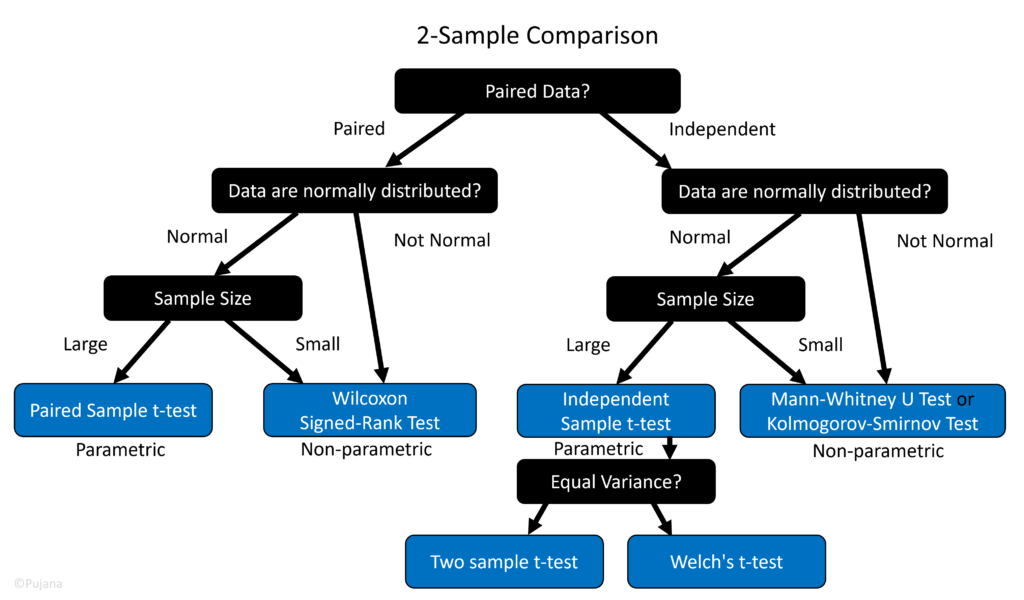Select Page

Online Statistical Hypothesis Tests (2-Sample Tests)

List of statistical tests

• Normality Tests: Shapiro–Wilk Test
• Levene’s Test for Homogeneity of Variance (test for the equality of variances)
• Paired Samples Tests: Paired Samples t-Test, Wilcoxon Signed-Rank Test
• Independent Samples Tests: Independent Samples t-Test, Mann–Whitney U Test, Kolmogorov-Smirnov Test

Decision TreeTool

Guides

• Choose either Pair or Independent test: if each row of data on two text areas come from the same subject, then your data is paired samples, else independent samples
• In case of Paired Test, see Normality Test results, there are two options.
• It is suggested to go with the non-parametric test if any normality test results suggest to use it. This tends to result in a higher p-value, thus less likelihood to detect a significant difference. On the other hand, parametric tests usually have more statistical power than non-parametric tests—it is more likely to detect a significant effect when one truly exists.
• In case of Independent Test, see Normality Test results.
• If a parametric test (t-test) is recommended, see Levene’s test for Homogeneity of Variance, to determine which result should be used (equal or unequal variance)
• If a non-parametric test is recommended, either Mann–Whitney U Test or Kolmogorov-Smirnov can be used. Different fields prefer different tests, but they should lead to the same conclusion in most cases.
• ※ For independent samples, if you know a population SD (or Variance), and the sample size is > 30, z-test is another option (not available in this tool). z-test tend to be more potent than t-test in terms of statistical power with large sample sizes. Nevertheless, in real life, we usually don’t know the population SD.
• Large or Small sample size is subjective: some books suggest that parametric should be used when n > 20

More Details